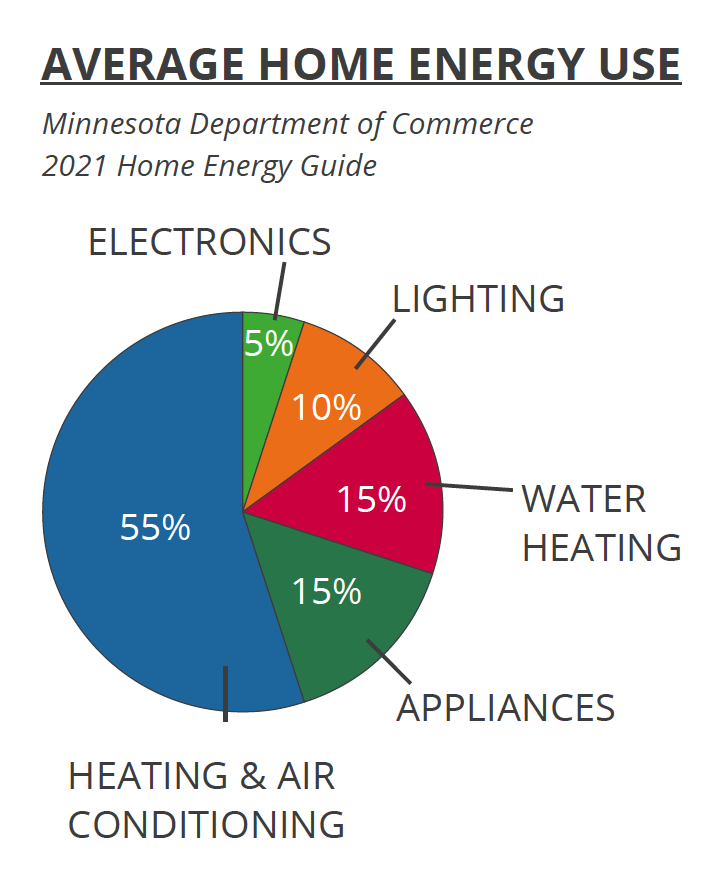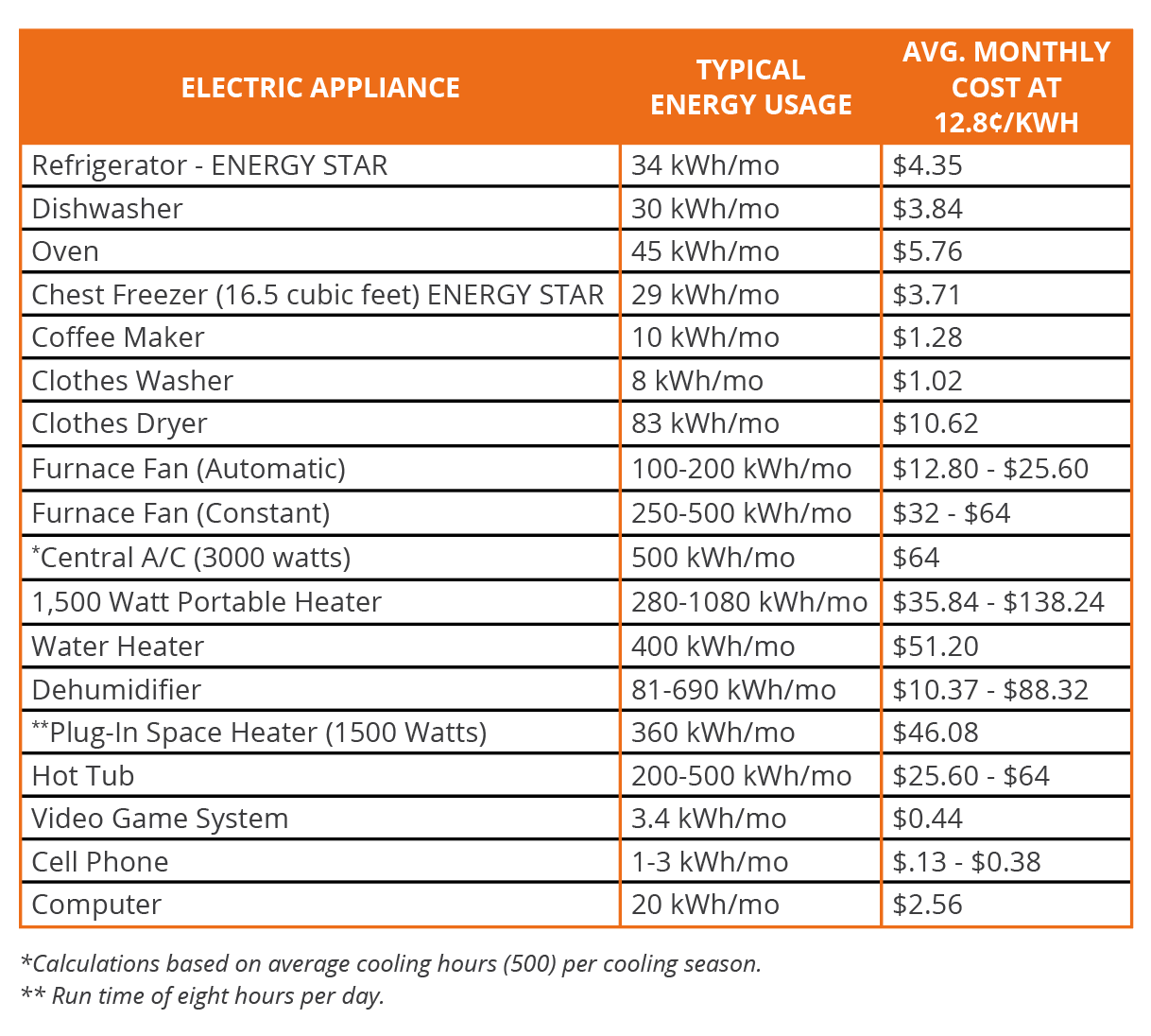This post contains outdated information and is kept for archived purposes only.

# Estimating Electricity Use and Cost

Step 1: Your electric bill is determined by the number of kilowatt-hours (kWh) used during a billing period. Your average kWh cost equals the dollar amount of the energy portions of your electric bill, divided by kWh used. \$96 ÷ 750 kWh = 12.8¢ per kWh.

Step 2: Wattage determines electrical use per hour and can be found on the serial plate of the appliance. Electric load may also be expressed in volts and amps, rather than watts. If so, multiply volts times amperes to determine the wattage. 120 volts x 12.1 amps = 1,452 watts.

Step 3: Use the formula shown in the following example to estimate use and cost. A light uses 100 watts and is left on for 15 hours. How many kWh are used and what does it cost you? kWh used = (100 watts x 15 hours) ÷ 1,000 watts = 1.5 kWh. Your cost = 1.5 kWh x 12.8¢ = 19.2¢.Figures are based on the average use of an appliance in good working condition.

Actual use will vary based on the number of hours used and the age and condition of equipment.